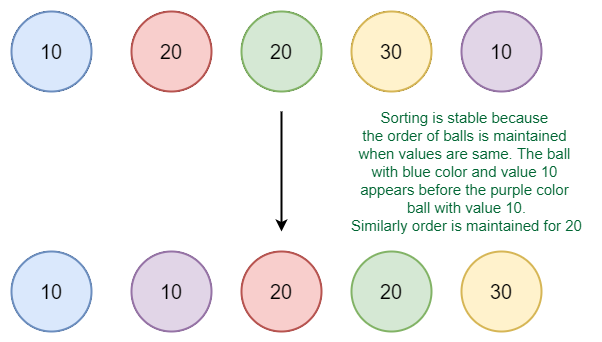GeeksforGeeks App
Open AppBrowser
Continue

# Stable and Unstable Sorting Algorithms

Stability is mainly essential when we have key-value pairs with duplicate keys possible (like people’s names as keys and their details as values). And we wish to sort these objects by keys.

## What is a stable sorting algorithm?

A sorting algorithm is said to be stable if two objects with equal keys appear in the same order in sorted output as they appear in the input data set

Formally stability may be defined as, how the algorithm treats equal elements. Let A[] be an array, and let ‘<‘ be a strict weak ordering on the elements of A[].  A sorting algorithm is stable if:whereis the sorting permutation ( sorting movesto position) .

Informally, stability means that equivalent elements retain their relative positions, after sorting.Example of stable sort

## Do we care for simple arrays like the array of integers?

When equal elements are indistinguishable, such as with integers, or more generally, any data where the entire element is the key, stability is not an issue. Stability is also not an issue if all keys are different.

## Where stable sorting algorithms are useful?

Consider the following dataset of Student Names and their respective class sections.If we sort this data according to name only, then it is highly unlikely that the resulting dataset will be grouped according to sections as well.So we might have to sort again to obtain the list of students section-wise too. But in doing so, if the sorting algorithm is not stable, we might get a result like this:The dataset is now sorted according to sections, but not according to names. In the name-sorted dataset, the tuple (Alice, B) was before (Eric, B) , but since the sorting algorithm is not stable, the relative order is lost. If on the other hand, we used a stable sorting algorithm, the result would be:Here the relative order between different tuples is maintained. It may be the case that the relative order is maintained in an Unstable Sort but that is highly unlikely.

## Which sorting algorithms are stable?

Some Sorting Algorithms are stable by nature, such as Bubble Sort, Insertion Sort, Merge Sort, Count Sort, etc. Comparison-based stable sorts such as Merge Sort and Insertion Sort maintain stability by ensuring that Elementcomes beforeif and only if, here i, j are indices, and. The relative order is preserved ifi.e.comes beforeOther non-comparison-based sorts such as Counting Sort maintain stability by ensuring that the Sorted Array is filled in reverse order so that elements with equivalent keys have the same relative position. Some sorts such as Radix Sort depend on another sort, with the only requirement that the other sort should be stable.

## Which sorting algorithms are unstable?

Quick Sort, Heap Sort etc., can be made stable by also taking the position of the elements into consideration. This change may be done in a way that does not compromise a lot on the performance and takes some extra space, possibly.

## Can we make any sorting algorithm stable?

Any given sorting algorithm which is not stable can be modified to be stable. There can be algorithm-specific ways to make it stable, but in general, any comparison-based sorting algorithm which is not stable by nature can be modified to be stable by changing the key comparison operation so that the comparison of two keys considers position as a factor for objects with equal keys.